# Circular Orbit

Gravity supplies the necessary centripetal force to hold a satellite in orbit about the earth. The circular orbit is a special case since orbits are generally ellipses, or hyperbolas in the case of objects which are merely deflected by the planet's gravity but not captured. Setting the gravity force from the universal law of gravity equal to the required centripetal force yields the description of the orbit. The orbit can be expressed in terms of the acceleration of gravity at the orbit.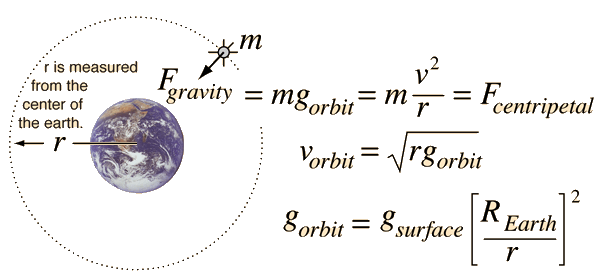The force of gravity in keeping an object in circular motion is an example of centripetal force. Since it acts always perpendicular to the motion, gravity does not do work on the orbiting object if it is in a circular orbit.

### Calculation

Index

Gravity concepts

Orbit concepts

 HyperPhysics***** Mechanics ***** Rotation R Nave
Go Back

# Earth's Gravity

The weight of an object is given by W=mg, the force of gravity, which comes from the law of gravity at the surface of the Earth in the inverse square law form:At standard sea level, the acceleration of gravity has the value g = 9.8 m/s2, but that value diminishes according to the inverse square law at greater distances from the earth. The value of g at any given height, say the height of an orbit, can be calculated from the above expression.

Above the earth's surface at a height of h =m = x 106 m, which corresponds to a radius r = x earth radius, the acceleration of gravity is g = m/s2 = x g on the earth's surface.

Please note that the above calculation gives the correct value for the acceleration of gravity only for positive values of h, i.e., for points outside the Earth. If you drilled a hole through the center of the Earth, the acceleration of gravity would decrease with the radius on the way to the center of the Earth. If the Earth were of uniform density (which it is not!), the acceleration of gravity would decrease linearly to half the surface value of g at half the radius of the Earth and approach zero as you approached the center of the Earth.

 Hole through center of Earth
Index

Gravity concepts

Orbit concepts

 HyperPhysics***** Mechanics ***** Rotation R Nave
Go Back

# Binary Circular Orbit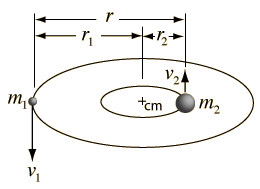From the gravity force and the necessary centripetal force: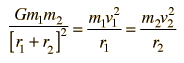If you are riding on one of the masses, the relative motion equation has the same form if you substitute the reduced mass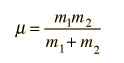which gives the orbit equation: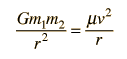This leads to Kepler's 3rd law (the Law of Periods) which is useful for the analysis of the orbits of moons and binary stars.

Since the period T of the orbit is given by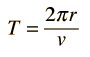then the motion equation can be written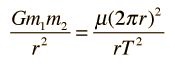which reduces to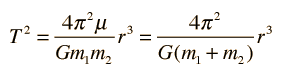If we use the convenient astronomical units

• r = a in AU (astronomical units)
• G = 4π2 m in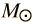(solar masses)
• T in years
then this takes the form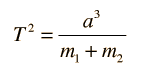This applies to circular orbits where a is the radius, and to elliptical orbits where a is the semi-major axis.
and from just the period and orbit radius you can obtain the sum of the masses m1 + m2. If you can obtain the individual orbit radii r1 and r2 then you can use the center of mass conditionwith the measured mass sum to obtain the individual masses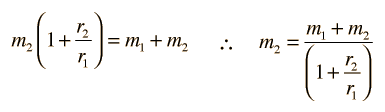These relationships are important in the study of visual binaries.
Index

Gravity concepts

Orbit concepts

 HyperPhysics***** Mechanics ***** Rotation R Nave
Go Back

# Reduced Mass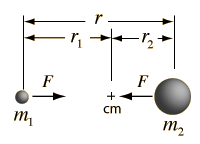The relative motion of two objects that are acted upon by a central force can be described by Newton's 2nd Law as if they were a single mass with a value called the "reduced mass".
 From Newton's 3rd Law: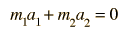The relative acceleration of the two masses is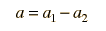Since a2 is negative, this can be rewritten in terms of the magnitudes of the quantities: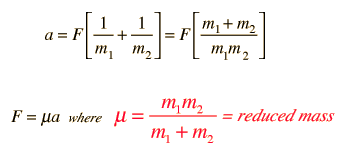Applications: Binary orbit Rotation of molecule H-D doublet
Index

Gravity concepts

 HyperPhysics***** Mechanics ***** Rotation R Nave
Go Back